# Limit-absorption principle

(diff) ← Older revision | Latest revision (diff) | Newer revision → (diff)

A manner for uniquely finding solutions to equations analogous to the Helmholtz equation by introducing an infinitesimal absorption. Mathematically the principle is as follows. Letbe an unbounded region in, letbe the self-adjoint operator on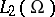given by the differential expression,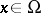, and homogeneous boundary conditions onand letbe a point in the continuous spectrum of. Then for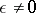the equation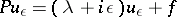is uniquely solvable in, and in certain cases it is possible to find solutionsof the equation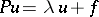by the limit transition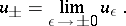It is assumed here thathas compact support and the convergence, as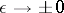, is understood in the sense of, where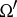is an arbitrary bounded set in. Sinceis a point of the continuous spectrum of, the limit indoes not exist, in general.

The first limit-absorption principle was formulated for the Helmholtz equation in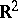(cf. ):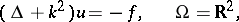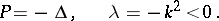The solutions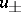found using this principle are diverging or converging waves and satisfy the radiation conditions at infinity. These results were carried over (cf. , ) to elliptic boundary value problems in the exterior of bounded regions infor an operator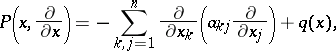(*)

where the coefficientstend to constants sufficiently rapidly as. In order that the limit-absorption principle holds in this case it is necessary thatis not an eigen value ofor thatis orthogonal to the eigen functions. A theorem of T. Kato (cf. ) gives sufficient conditions for the absence of eigen values in the continuous spectrum of the operator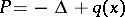. Such a theorem has been obtained for the operator (*) (cf. ). The limit-absorption principle has been substantiated for certain regions with non-compact boundary (cf. , ).

A limit-absorption principle and corresponding radiation conditions have been found for higher-order equations and for systems of equations (cf. ); they consist of the following. Let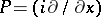be an elliptic (or hypo-elliptic) operator satisfying: 1) the polynomial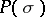has real coefficients; 2) the surface,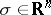, decomposes into connected smooth surfaces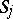,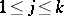, whose curvatures do not vanish; and 3)on. Suppose that an orientation is given on, i.e. for each surface one has independently chosen a normal direction. Let, let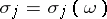be a point onat whichandhave identical direction and let. Then the function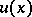does satisfy the radiation conditions if it can be represented as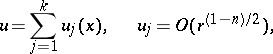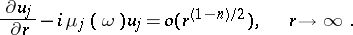These conditions determine a unique solution of the equation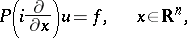for any functionwith compact support. The limit-absorption principle for this equation is that this solution can be obtained as the limit, for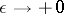, of the unique solutionof the elliptic equation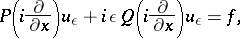where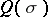has real coefficients and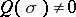on. Depending on the choice of,, one obtains in the limit solutions satisfying the radiation conditions corresponding to some orientation of. This principle has been substantiated for higher-order equations and systems with variable coefficients in the exterior of bounded regions (cf. ), as well as in the case of non-convex. For such equations there is also a uniqueness theorem of Kato type.

How to Cite This Entry:
Limit-absorption principle. Encyclopedia of Mathematics. URL: http://encyclopediaofmath.org/index.php?title=Limit-absorption_principle&oldid=13580
This article was adapted from an original article by B.R. Vainberg (originator), which appeared in Encyclopedia of Mathematics - ISBN 1402006098. See original article